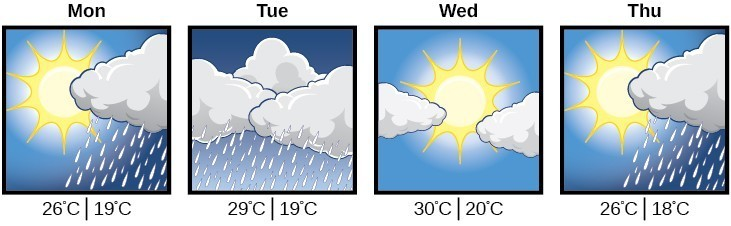## Verify inverse functions

Suppose a fashion designer traveling to Milan for a fashion show wants to know what the temperature will be. He is not familiar with the Celsius scale. To get an idea of how temperature measurements are related, he asks his assistant, Betty, to convert 75 degrees Fahrenheit to degrees Celsius. She finds the formula

$C=\frac{5}{9}\left(F - 32\right)$

and substitutes 75 for $F$ to calculate

$\frac{5}{9}\left(75 - 32\right)\approx {24}^{ \circ} {C}$.Figure 2

Knowing that a comfortable 75 degrees Fahrenheit is about 24 degrees Celsius, he sends his assistant the week’s weather forecast for Milan, and asks her to convert all of the temperatures to degrees Fahrenheit.

At first, Betty considers using the formula she has already found to complete the conversions. After all, she knows her algebra, and can easily solve the equation for $F$ after substituting a value for $C$. For example, to convert 26 degrees Celsius, she could write

$\begin{cases}26=\frac{5}{9}\left(F - 32\right)\hfill \\ 26\cdot \frac{9}{5}=F - 32\hfill \\ F=26\cdot \frac{9}{5}+32\approx 79\hfill \end{cases}$

After considering this option for a moment, however, she realizes that solving the equation for each of the temperatures will be awfully tedious. She realizes that since evaluation is easier than solving, it would be much more convenient to have a different formula, one that takes the Celsius temperature and outputs the Fahrenheit temperature.

The formula for which Betty is searching corresponds to the idea of an inverse function, which is a function for which the input of the original function becomes the output of the inverse function and the output of the original function becomes the input of the inverse function.

Given a function $f\left(x\right)$, we represent its inverse as ${f}^{-1}\left(x\right)$, read as $f$ inverse of $x.\text{}$ The raised $-1$ is part of the notation. It is not an exponent; it does not imply a power of $-1$ . In other words, ${f}^{-1}\left(x\right)$ does not mean $\frac{1}{f\left(x\right)}$ because $\frac{1}{f\left(x\right)}$ is the reciprocal of $f$ and not the inverse.

The “exponent-like” notation comes from an analogy between function composition and multiplication: just as ${a}^{-1}a=1$ (1 is the identity element for multiplication) for any nonzero number $a$, so ${f}^{-1}\circ f\\$ equals the identity function, that is,

$\left({f}^{-1}\circ f\right)\left(x\right)={f}^{-1}\left(f\left(x\right)\right)={f}^{-1}\left(y\right)=x\\$

This holds for all $x$ in the domain of $f$. Informally, this means that inverse functions “undo” each other. However, just as zero does not have a reciprocal, some functions do not have inverses.

Given a function $f\left(x\right)$, we can verify whether some other function $g\left(x\right)$ is the inverse of $f\left(x\right)$ by checking whether either $g\left(f\left(x\right)\right)=x$ or $f\left(g\left(x\right)\right)=x$ is true. We can test whichever equation is more convenient to work with because they are logically equivalent (that is, if one is true, then so is the other.)

For example, $y=4x$ and $y=\frac{1}{4}x\\$ are inverse functions.

$\left({f}^{-1}\circ f\right)\left(x\right)={f}^{-1}\left(4x\right)=\frac{1}{4}\left(4x\right)=x\\$

and

$\left({f}^{}\circ {f}^{-1}\right)\left(x\right)=f\left(\frac{1}{4}x\right)=4\left(\frac{1}{4}x\right)=x\\$

A few coordinate pairs from the graph of the function $y=4x$ are (−2, −8), (0, 0), and (2, 8). A few coordinate pairs from the graph of the function $y=\frac{1}{4}x\\$ are (−8, −2), (0, 0), and (8, 2). If we interchange the input and output of each coordinate pair of a function, the interchanged coordinate pairs would appear on the graph of the inverse function.

### A General Note: Inverse Function

For any one-to-one function $f\left(x\right)=y$, a function ${f}^{-1}\left(x\right)$ is an inverse function of $f$ if ${f}^{-1}\left(y\right)=x$. This can also be written as ${f}^{-1}\left(f\left(x\right)\right)=x$ for all $x$ in the domain of $f$. It also follows that $f\left({f}^{-1}\left(x\right)\right)=x$ for all $x$ in the domain of ${f}^{-1}$ if ${f}^{-1}$ is the inverse of $f$.

The notation ${f}^{-1}$ is read $\text{}f$ inverse.” Like any other function, we can use any variable name as the input for ${f}^{-1}$, so we will often write ${f}^{-1}\left(x\right)$, which we read as $f$ inverse of $x.''$
Keep in mind that

${f}^{-1}\left(x\right)\ne \frac{1}{f\left(x\right)}$

and not all functions have inverses.

### Example 1: Identifying an Inverse Function for a Given Input-Output Pair

If for a particular one-to-one function $f\left(2\right)=4$ and $f\left(5\right)=12$, what are the corresponding input and output values for the inverse function?

### Solution

The inverse function reverses the input and output quantities, so if

$\begin{cases}{c}f\left(2\right)=4,\text{ then }{f}^{-1}\left(4\right)=2;\\ f\left(5\right)=12,{\text{ then f}}^{-1}\left(12\right)=5.\end{cases}$

Alternatively, if we want to name the inverse function $g$, then $g\left(4\right)=2$ and $g\left(12\right)=5$.

### Analysis of the Solution

Notice that if we show the coordinate pairs in a table form, the input and output are clearly reversed.

$\left(x,f\left(x\right)\right)$ $\left(x,g\left(x\right)\right)$
$\left(2,4\right)$ $\left(4,2\right)$
$\left(5,12\right)$ $\left(12,5\right)$

### Try It 1

Given that ${h}^{-1}\left(6\right)=2$, what are the corresponding input and output values of the original function $h?$

Solution

### How To: Given two functions $f\left(x\right)\\$ and $g\left(x\right)\\$, test whether the functions are inverses of each other.

1. Determine whether $f\left(g\left(x\right)\right)=x$ or $g\left(f\left(x\right)\right)=x$.
2. If either statement is true, then both are true, and $g={f}^{-1}$ and $f={g}^{-1}$. If either statement is false, then both are false, and $g\ne {f}^{-1}$ and $f\ne {g}^{-1}$.

### Example 2: Testing Inverse Relationships Algebraically

If $f\left(x\right)=\frac{1}{x+2}$ and $g\left(x\right)=\frac{1}{x}-2$, is $g={f}^{-1}?$

### Solution

$\begin{cases} g\left(f\left(x\right)\right)=\frac{1}{\left(\frac{1}{x+2}\right)}{-2 }\hfill\\={ x }+{ 2 } -{ 2 }\hfill\\={ x }\hfill \end{cases}\\$

so

$g={f}^{-1}\text{ and }f={g}^{-1}$

This is enough to answer yes to the question, but we can also verify the other formula.

$\begin{cases} f\left(g\left(x\right)\right)=\frac{1}{\frac{1}{x}-2+2}\\ =\frac{1}{\frac{1}{x}}\hfill \\ =x\hfill \end{cases}\\$

### Analysis of the Solution

Notice the inverse operations are in reverse order of the operations from the original function.

### Try It 2

If $f\left(x\right)={x}^{3}-4$ and $g\left(x\right)=\sqrt{x+4}$, is $g={f}^{-1}?$

Solution

### Example 3: Determining Inverse Relationships for Power Functions

If $f\left(x\right)={x}^{3}$ (the cube function) and $g\left(x\right)=\frac{1}{3}x$, is $g={f}^{-1}?\\$

### Solution

$f\left(g\left(x\right)\right)=\frac{{x}^{3}}{27}\ne x\\$

No, the functions are not inverses.

### Analysis of the Solution

The correct inverse to the cube is, of course, the cube root $\sqrt{x}={x}^{\frac{1}{3}}\\$, that is, the one-third is an exponent, not a multiplier.

### Try It 3

If $f\left(x\right)={\left(x - 1\right)}^{3}\text{and}g\left(x\right)=\sqrt{x}+1\\$, is $g={f}^{-1}?\\$

Solution